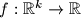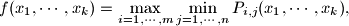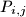### IMO Shortlist 2012 problem A7

Kvaliteta:
Avg: 0,0
Težina:
Avg: 9,0
We say that a function$f:\mathbb{R}^k \rightarrow \mathbb{R}$ is a metapolynomial if, for some positive integer$m$ and$n$, it can be represented in the formwhere$P_{i,j}$ are multivariate polynomials. Prove that the product of two metapolynomials is also a metapolynomial.
Izvor: Međunarodna matematička olimpijada, shortlist 2012